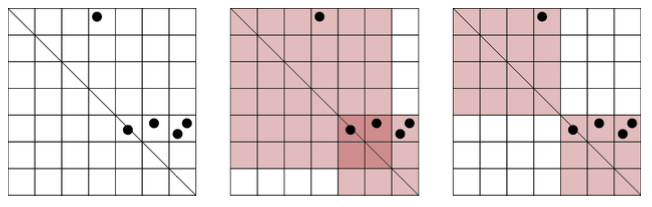# #240. 【IOI2016】aliens

• 拍摄的区域必须是正方形。
• 这个正方形的两个对角（注：变通理解为主对角线）全部包含在网格的主对角线中。
• 网格中的每个小方格或者完全在拍摄范围内，或者完全在拍摄范围外。

• 每个包含至少一个兴趣点的小方格必须被至少拍摄到一次，并且
• 被拍摄到至少一次的小方格数目必须是最小的。

### 实现细节

• long long take_photos(int n, int m, int k, std::vector<int> r, std::vector<int> c)
• $n$：兴趣点的数目，
• $m$：网格中的行数（也是列数），
• $k$：卫星能够拍摄高分辨率照片的最大次数，
• $r$ 和 $c$：两个长度为 $n$ 的数组，描述网格中包含兴趣点的那些小方格的坐标。对于 $0 \le i \le n - 1$，第 $i$ 个兴趣点位于坐标为 $(r_i, c_i)$ 的小方格，
• 这个函数应该返回被至少拍摄一次的小方格的总数的最小值（这些照片必须覆盖所有兴趣点）。

### 样例一

take_photos(5, 7, 2, [0, 4, 4, 4, 4], [3, 4, 6, 5, 6])### 样例二

take_photos(2, 6, 2, [1, 4], [4, 1])### 子任务

14$50$$100$$k=n$
212$500$$1000$$r_i = c_i$
39$500$$1000 4164000$$1000000$
519$50000$$1000000$$k \le 100$
640$100000$$1000000$

### 评测方式

• 第 $1$ 行：整数 $n, m$ 和 $k$，
• 第 $2 + i~(0 \le i \le n - 1)$ 行：整数 $r_i$ 和 $c_i$。